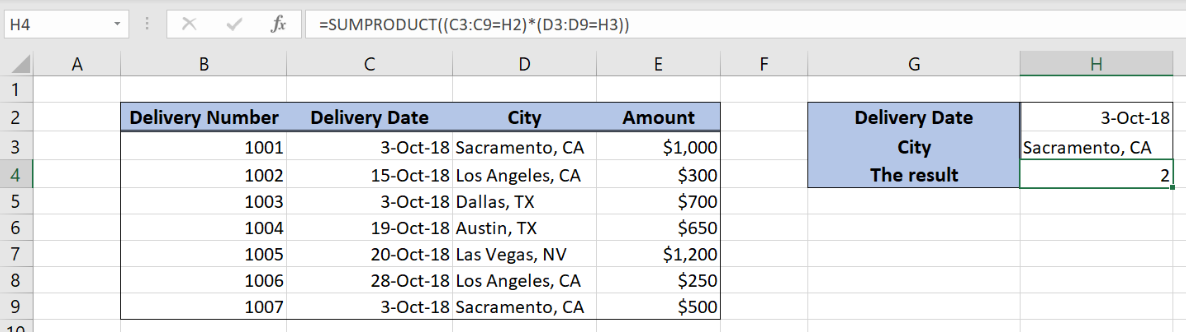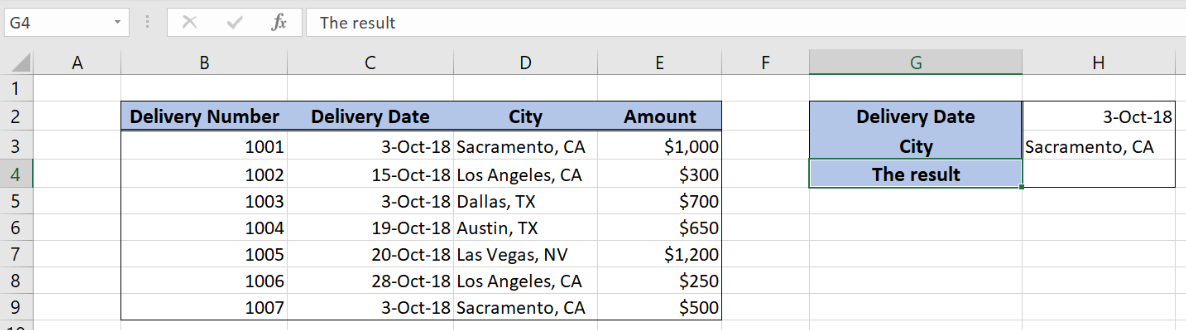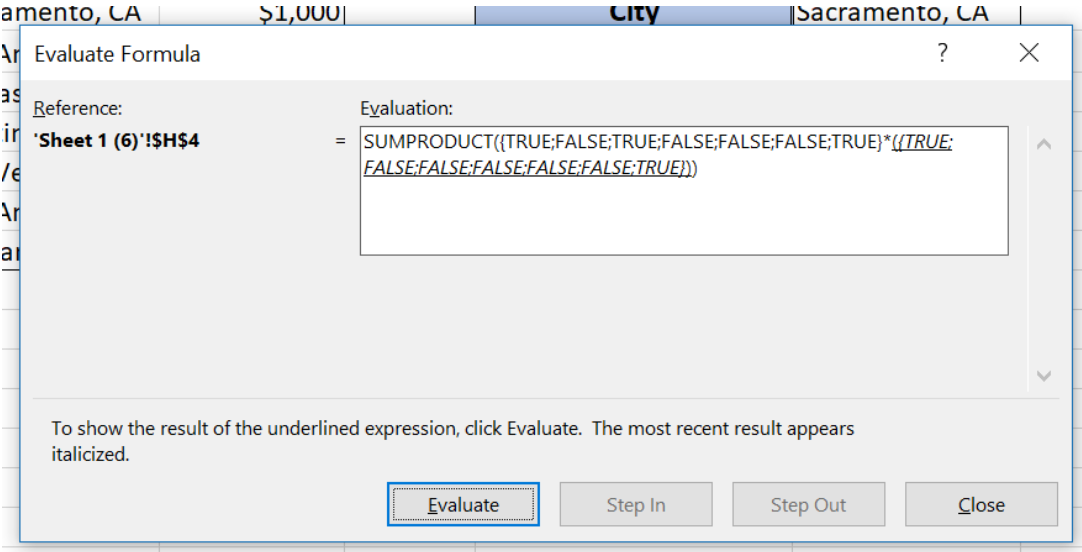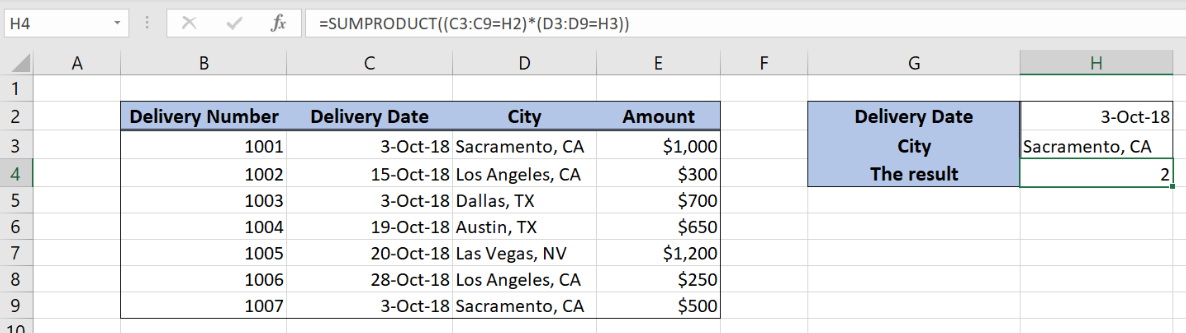Get instant live expert help with Excel or Google Sheets“My Excelchat expert helped me in less than 20 minutes, saving me what would have been 5 hours of work!”

#### Post your problem and you’ll get Expert help in seconds.

Your message must be at least 40 characters
Our professional Expert are available now. Your privacy is guaranteed.

# Count if row meets multiple internal criteria

Excel allows a user to count rows if multiple criteria are met by using the SUMPRODUCT function. This step by step tutorial will assist all levels of Excel users in counting rows that meet multiple criteria.Figure 1. The result of the SUMPRODUCT function

## Syntax of the SUMPRODUCT Formula

The generic formula for the SUMPRODUCT function is:

`=SUMPRODUCT((array1 = condition1) * (array2 = condition2))`

The parameters of the SUMPRODUCT function are:

• array1 and array2 – range of cells where we want to apply a condition
• condition1 and condition2 – conditions which we want to apply on arrays.

The function compares cells one by one and returns Booleans TRUE or FALSE for both arrays. In the end, it multiplies Booleans and sums the results.

## Setting up Our Data for the SUMPRODUCT FunctionFigure 2. Data that we will use in the SUMPRODUCT example

Let’s look at the structure of the data we will use. The table consists of 4 columns: “Delivery Number” (column B), “Delivery Date” (column C), “City” (column D) and “Amount” (column E). In the cell H4, we want to get the count of all the rows that meet two conditions. The first condition is Delivery Date equal to H2, while the second is City equal to H3.

## Using the SUMPRODUCT to Count Rows Meeting Two Criteria

In our example, we want to count all rows where the delivery date is 3-Oct-18 and City is Sacramento, CA.

The formula looks like:

`=SUMPRODUCT((C3:C9 = H2) * (D3:D9 = H3))`

The array1 is C3:C9 and condition1 is H2. The array2 is D3:D9 and condition2 is H3. Let’s evaluate the formula and see the result:Figure 3. Evaluate the SUMPRODUCT function

As you can see, the function has TRUE if conditions are met or FALSE if not. The first part of the function returns {TRUE; FALSE; TRUE; FALSE; FALSE; FALSE; TRUE}. The second part returns {TRUE; FALSE; FALSE; FALSE; FALSE; FALSE; TRUE}.

After this multiplies these values one by one, where TRUE is 1 AND FALSE 0. The result of the multiplication is: {1, 0, 0, 0, 0, 0, 1}. In the end, all the values are summed and the result in the cell H4 is 2.

To apply the SUMPRODUCT function, we need to follow these steps:

• Select cell H4 and click on it
• Insert the formula: `=SUMPRODUCT((C3:C9 = H2) * (D3:D9 = H3))`
• Press enterFigure 4. Using the SUMPRODUCT to count rows meeting two criteria

Most of the time, the problem you will need to solve will be more complex than a simple application of a formula or function. If you want to save hours of research and frustration, try our live Excelchat service! Our Excel Experts are available 24/7 to answer any Excel question you may have. We guarantee a connection within 30 seconds and a customized solution within 20 minutes.

### Did this post not answer your question? Get a solution from connecting with the expert.Another blog reader asked this question today on Excelchat:
Solution examplesYes hello Im looking for help on conditional formatting I currently have a conditional format for D9>D32 and so on from D9:S20. I need to add an if statement that references cell D4 if the first four characters are 1358 for the rule to apply can you please assist?
Solved by Z. U. in 20 minsUse the Vlookup Function to complete the "employee" column of table 2. Use "job Id" from table 2 as your lookup_value(s) and table 1 as your reference.
Solved by C. H. in 16 minsI have a list or people who are ranked in numbers from 3 to 6 I need to recognize the contents of each multiple cells and create an equivalent letter. for that value into another column. e.g. Cell E1 = 3 to show in new cell that row (H1) the letter "C" I have multiple rows with different values in column "E" Can this be done?
Solved by I. J. in 30 minsIf a cell in another sheet is populated I need a vlookup done. If the cell is not populated I need the cell to return blank.
Solved by T. D. in 60 minsI am trying to make a chart that turns a week range red if nothing is entered in the range. If something is entered then I would like it to turn green. Please Help
Solved by E. U. in 43 mins## Subscribe to Excelchat.coAnother blog reader asked this question today on Excelchat: